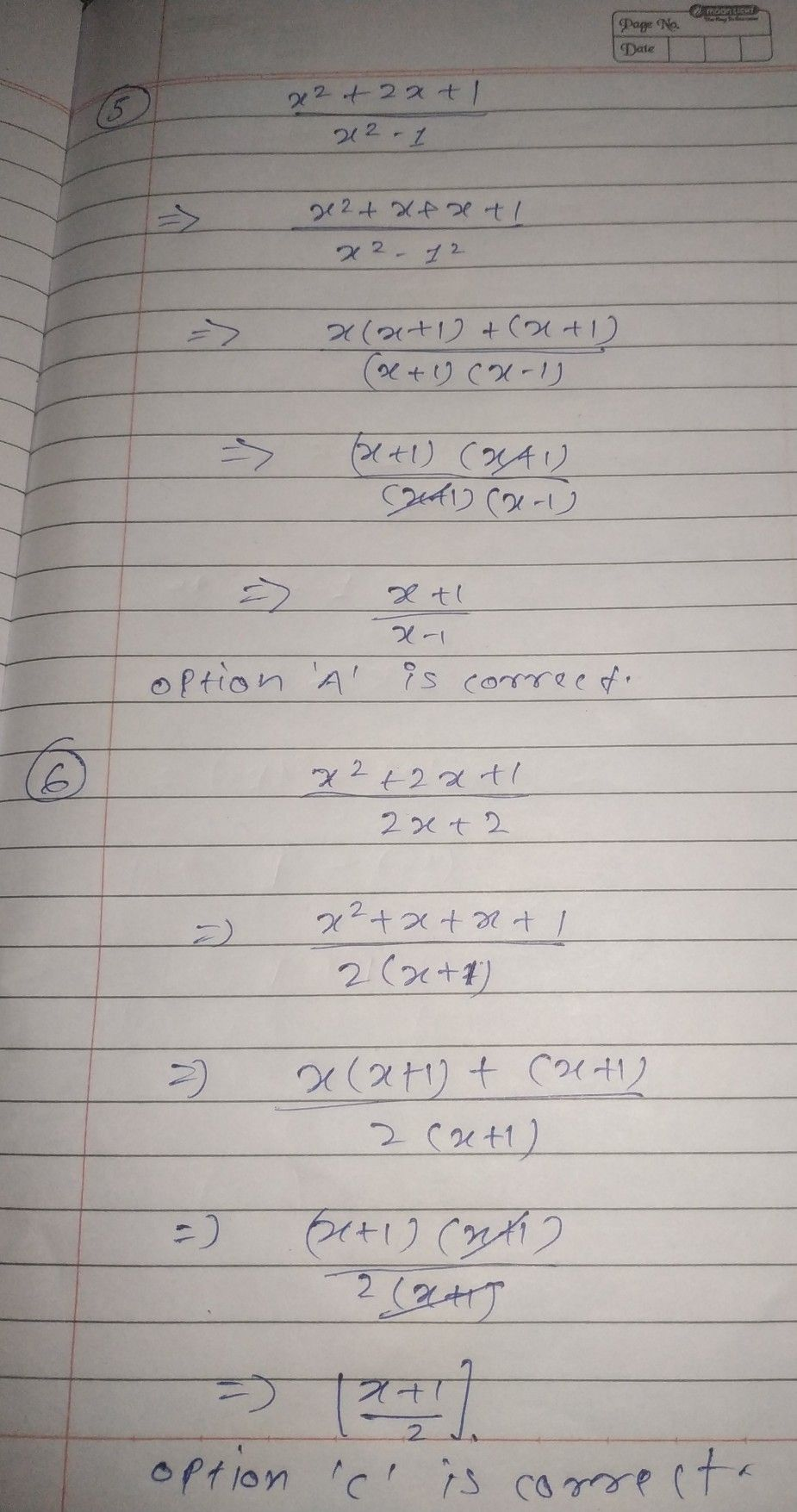Symbol
Problem$5$ Which of the following is the $simD|es1$ form of $\dfrac {x^{2}+2x+1} {x^{2-1}}7$ $A$ $x-1$ $x+1$ $-$ B. $\dfrac {x+1} {1-x}$ $D$ $\dfrac {1-x} {x+1}$ $\dfrac {x-1} {x+1}$ $6.$ Which of the following rational expression has $\dfrac {x+1} {2}$ as simplest form? $A$ $\dfrac {2x+1} {4}$ $C$ $\dfrac {x^{2}+2x+1} {2x+2}$ B. $\dfrac {x^{2}-1} {2x+2}$ . D$\dfrac {\left(x+1\right)\left(x+2\right)} {2x+2}$
7th-9th grade
Algebra
Search count: 105
SolutionQanda teacher - shadabStudent
thx po